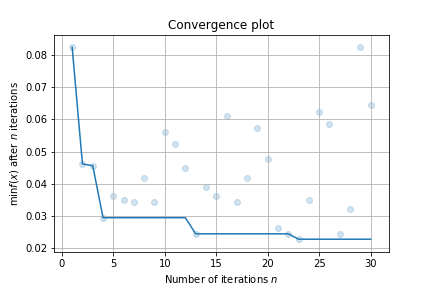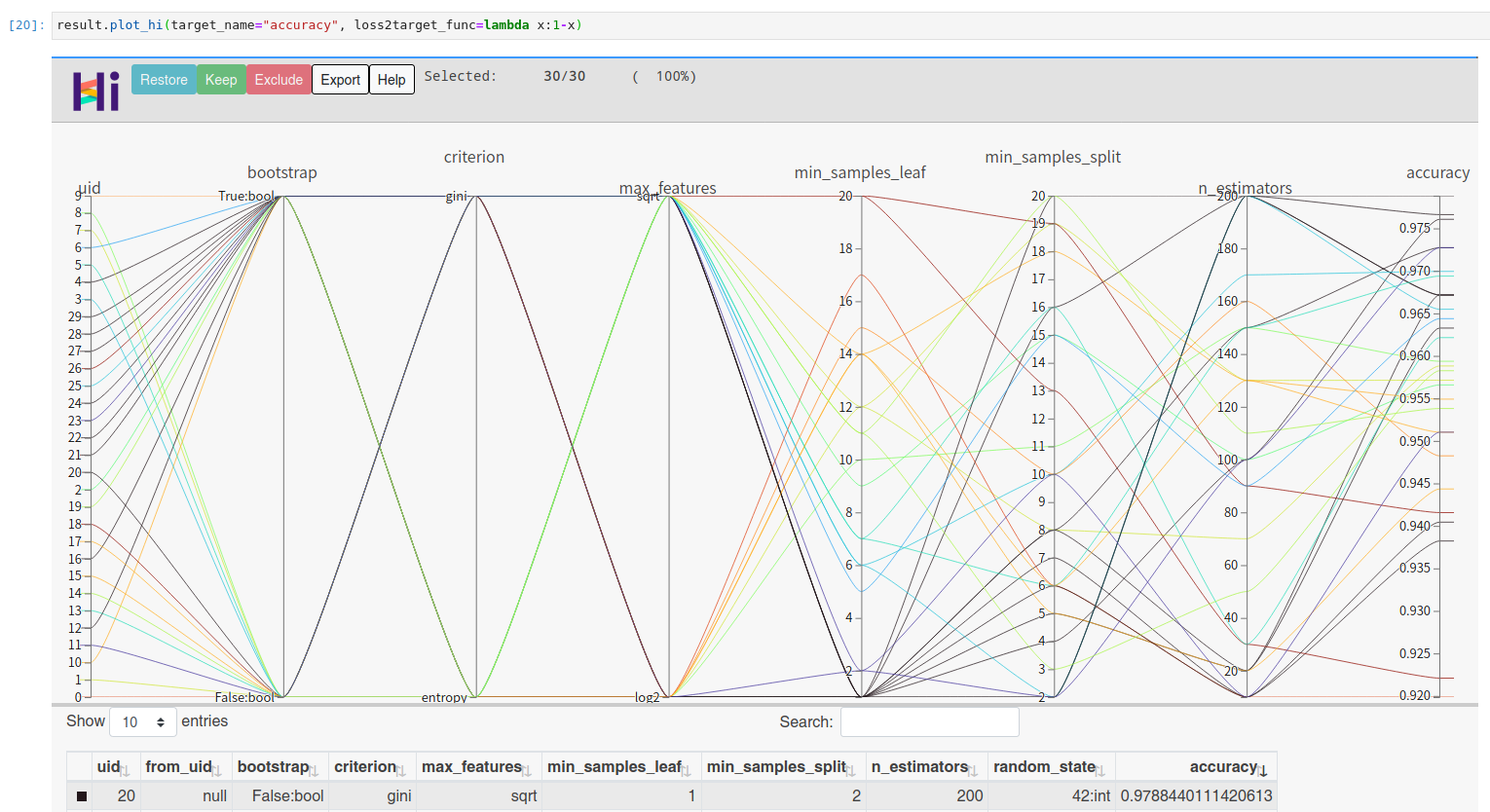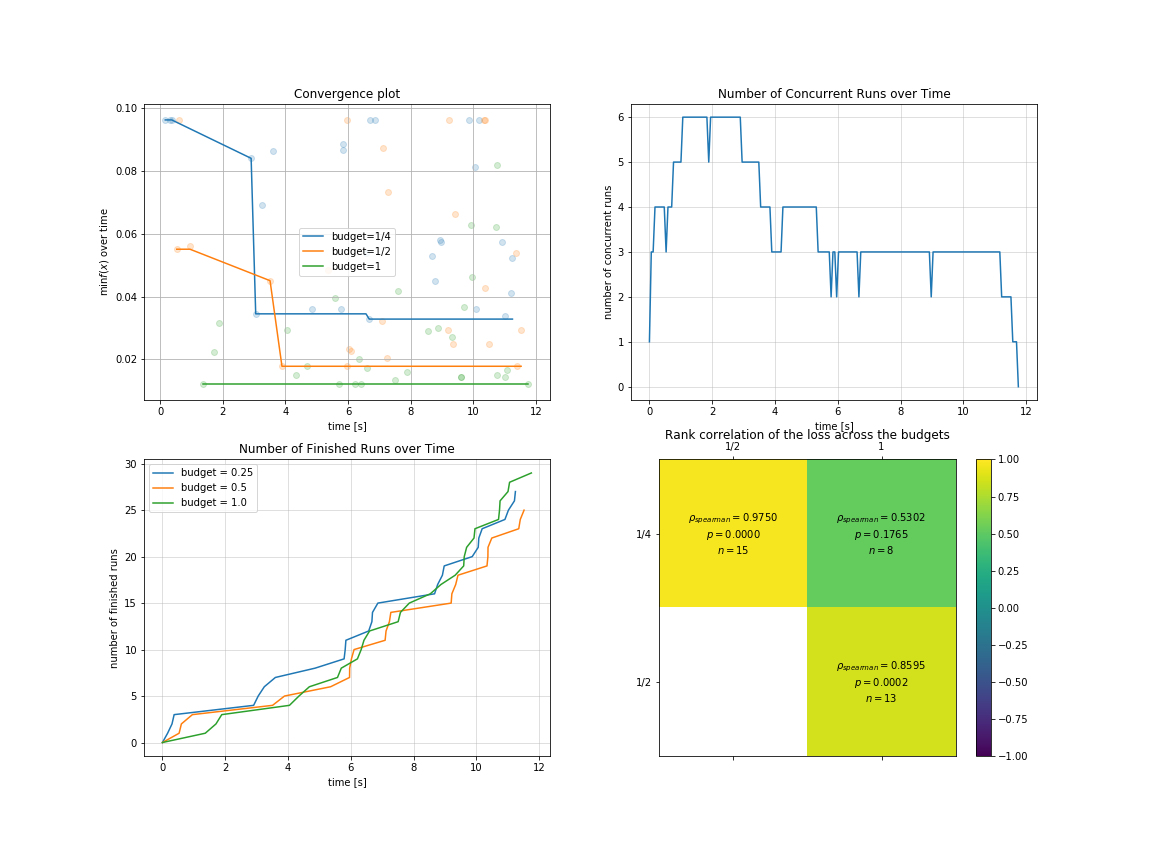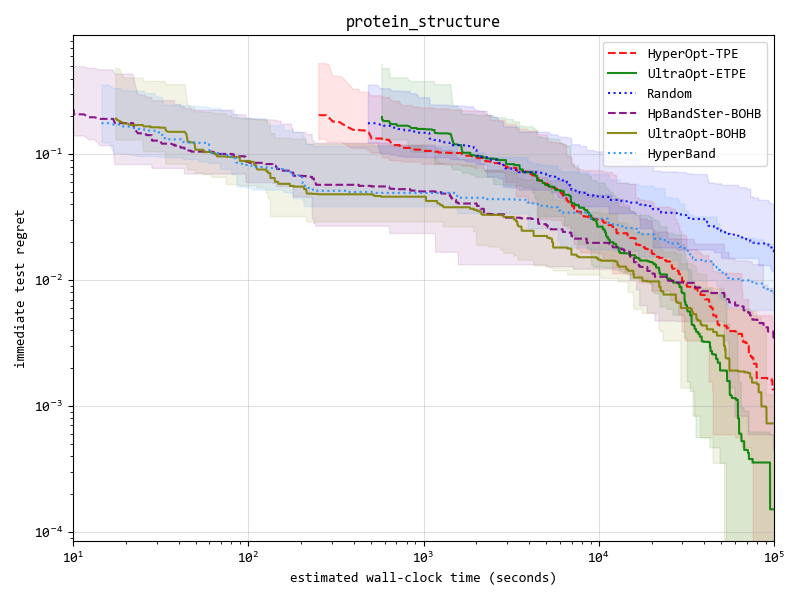# Distributed Asynchronous Hyperparameter Optimization better than HyperOpt. 比HyperOpt更强的分布式异步超参优化库。auto-flow, updated 🕥 2022-01-22 09:06:59`UltraOpt` : Distributed Asynchronous Hyperparameter Optimization better than HyperOpt.

`UltraOpt` is a simple and efficient library to minimize expensive and noisy black-box functions, it can be used in many fields, such as HyperParameter Optimization(HPO) and Automatic Machine Learning(AutoML).

After absorbing the advantages of existing optimization libraries such as HyperOpt, SMAC3, scikit-optimize and HpBandSter, we develop `UltraOpt` , which implement a new bayesian optimization algorithm : Embedding-Tree-Parzen-Estimator(ETPE), which is better than HyperOpt' TPE algorithm in our experiments. Besides, The optimizer of `UltraOpt` is redesigned to adapt HyperBand & SuccessiveHalving Evaluation Strategies and MapReduce & Async Communication Conditions. Finally, you can visualize `Config Space` and `optimization process & results` by `UltraOpt`'s tool function. Enjoy it !

• Documentation

• Tutorials

# Installation

UltraOpt requires Python 3.6 or higher.

You can install the latest release by `pip`:

```bash pip install ultraopt```

```bash git clone https://github.com/auto-flow/ultraopt.git && cd ultraopt python setup.py install```

# Quick Start

## Using UltraOpt in HPO

Let's learn what `UltraOpt` doing with several examples (you can try it on your `Jupyter Notebook`).

You can learn Basic-Tutorial in here, and `HDL`'s Definition in here.

Before starting a black box optimization task, you need to provide two things:

• parameter domain, or the Config Space
• objective function, accept `config` (`config` is sampled from Config Space), return `loss`

Let's define a Random Forest's HPO Config Space by `UltraOpt`'s `HDL` (Hyperparameter Description Language):

```python HDL = { "n_estimators": {"_type": "int_quniform","_value": [10, 200, 10], "_default": 100}, "criterion": {"_type": "choice","_value": ["gini", "entropy"],"_default": "gini"}, "max_features": {"_type": "choice","_value": ["sqrt","log2"],"_default": "sqrt"}, "min_samples_split": {"_type": "int_uniform", "_value": [2, 20],"_default": 2}, "min_samples_leaf": {"_type": "int_uniform", "_value": [1, 20],"_default": 1}, "bootstrap": {"_type": "choice","_value": [True, False],"_default": True}, "random_state": 42 }```

And then define an objective function:

```python from sklearn.ensemble import RandomForestClassifier from sklearn.datasets import load_digits from sklearn.model_selection import cross_val_score, StratifiedKFold from ultraopt.hdl import layering_config X, y = load_digits(return_X_y=True) cv = StratifiedKFold(5, True, 0) def evaluate(config: dict) -> float: model = RandomForestClassifier(**layering_config(config)) return 1 - float(cross_val_score(model, X, y, cv=cv).mean())```

Now, we can start an optimization process:

```python from ultraopt import fmin result = fmin(eval_func=evaluate, config_space=HDL, optimizer="ETPE", n_iterations=30) result```

``` 100%|██████████| 30/30 [00:36<00:00, 1.23s/trial, best loss: 0.023]

+-----------------------------------+ | HyperParameters | Optimal Value | +-------------------+---------------+ | bootstrap | True:bool | | criterion | gini | | max_features | log2 | | min_samples_leaf | 1 | | min_samples_split | 2 | | n_estimators | 200 | +-------------------+---------------+ | Optimal Loss | 0.0228 | +-------------------+---------------+ | Num Configs | 30 | +-------------------+---------------+ ```

Finally, make a simple visualizaiton:

```python result.plot_convergence()```You can visualize high dimensional interaction by facebook's hiplot:

```python !pip install hiplot result.plot_hi(target_name="accuracy", loss2target_func=lambda x:1-x)```## Using UltraOpt in AutoML

Let's try a more complex example: solve AutoML's CASH Problem  (Combination problem of Algorithm Selection and Hyperparameter optimization) by BOHB algorithm (Combine HyperBand Evaluation Strategies with `UltraOpt`'s ETPE optimizer) .

You can learn Conditional Parameter and complex `HDL`'s Definition in here, AutoML implementation tutorial in here and Multi-Fidelity Optimization in here.

First of all, let's define a CASH `HDL` :

```python HDL = { 'classifier(choice)':{ "RandomForestClassifier": { "n_estimators": {"_type": "int_quniform","_value": [10, 200, 10], "_default": 100}, "criterion": {"_type": "choice","_value": ["gini", "entropy"],"_default": "gini"}, "max_features": {"_type": "choice","_value": ["sqrt","log2"],"_default": "sqrt"}, "min_samples_split": {"_type": "int_uniform", "_value": [2, 20],"_default": 2}, "min_samples_leaf": {"_type": "int_uniform", "_value": [1, 20],"_default": 1}, "bootstrap": {"_type": "choice","_value": [True, False],"_default": True}, "random_state": 42 }, "KNeighborsClassifier": { "n_neighbors": {"_type": "int_loguniform", "_value": [1,100],"_default": 3}, "weights" : {"_type": "choice", "_value": ["uniform", "distance"],"_default": "uniform"}, "p": {"_type": "choice", "_value": [1, 2],"_default": 2}, }, } }```

And then, define a objective function with an additional parameter `budget` to adapt to HyperBand evaluation strategy:

```python from sklearn.neighbors import KNeighborsClassifier import numpy as np def evaluate(config: dict, budget: float) -> float: layered_dict = layering_config(config) AS_HP = layered_dict['classifier'].copy() AS, HP = AS_HP.popitem() ML_model = eval(AS)(**HP) scores = [] for i, (train_ix, valid_ix) in enumerate(cv.split(X, y)): rng = np.random.RandomState(i) size = int(train_ix.size * budget) train_ix = rng.choice(train_ix, size, replace=False) X_train,y_train = X[train_ix, :],y[train_ix] X_valid,y_valid = X[valid_ix, :],y[valid_ix] ML_model.fit(X_train, y_train) scores.append(ML_model.score(X_valid, y_valid)) score = np.mean(scores) return 1 - score```

You should instance a `multi_fidelity_iter_generator` object for the purpose of using HyperBand Evaluation Strategy :

```python from ultraopt.multi_fidelity import HyperBandIterGenerator hb = HyperBandIterGenerator(min_budget=1/4, max_budget=1, eta=2) hb.get_table()```

iter 0 iter 1 iter 2
stage 0 stage 1 stage 2 stage 0 stage 1 stage 0
num_config 4 2 1 2 1 3
budget 1/4 1/2 1 1/2 1 1

let's combine HyperBand Evaluation Strategies with `UltraOpt`'s ETPE optimizer , and then start an optimization process:

```python result = fmin(eval_func=evaluate, config_space=HDL, optimizer="ETPE", # using bayesian optimizer: ETPE multi_fidelity_iter_generator=hb, # using HyperBand n_jobs=3, # 3 threads n_iterations=20) result```

``` 100%|██████████| 88/88 [00:11<00:00, 7.48trial/s, max budget: 1.0, best loss: 0.012]

+--------------------------------------------------------------------------------------------------------------------------+ | HyperParameters | Optimal Value | +-----------------------------------------------------+----------------------+----------------------+----------------------+ | classifier:choice | KNeighborsClassifier | KNeighborsClassifier | KNeighborsClassifier | | classifier:KNeighborsClassifier:n_neighbors | 4 | 1 | 3 | | classifier:KNeighborsClassifier:p | 2:int | 2:int | 2:int | | classifier:KNeighborsClassifier:weights | distance | uniform | uniform | | classifier:RandomForestClassifier:bootstrap | - | - | - | | classifier:RandomForestClassifier:criterion | - | - | - | | classifier:RandomForestClassifier:max_features | - | - | - | | classifier:RandomForestClassifier:min_samples_leaf | - | - | - | | classifier:RandomForestClassifier:min_samples_split | - | - | - | | classifier:RandomForestClassifier:n_estimators | - | - | - | | classifier:RandomForestClassifier:random_state | - | - | - | +-----------------------------------------------------+----------------------+----------------------+----------------------+ | Budgets | 1/4 | 1/2 | 1 (max) | +-----------------------------------------------------+----------------------+----------------------+----------------------+ | Optimal Loss | 0.0328 | 0.0178 | 0.0122 | +-----------------------------------------------------+----------------------+----------------------+----------------------+ | Num Configs | 28 | 28 | 32 | +-----------------------------------------------------+----------------------+----------------------+----------------------+ ```

You can visualize optimization process in `multi-fidelity` scenarios:

```python import pylab as plt plt.rcParams['figure.figsize'] = (16, 12) plt.subplot(2, 2, 1) result.plot_convergence_over_time(); plt.subplot(2, 2, 2) result.plot_concurrent_over_time(num_points=200); plt.subplot(2, 2, 3) result.plot_finished_over_time(); plt.subplot(2, 2, 4) result.plot_correlation_across_budgets();```## Advantage One: ETPE optimizer is more competitive

We implement 4 kinds of optimizers(listed in the table below), and `ETPE` optimizer is our original creation, which is proved to be better than other `TPE based optimizers` such as `HyperOpt's TPE` and `HpBandSter's BOHB` in our experiments.

Our experimental code is public available in here, experimental documentation can be found in here .

|Optimizer|Description| |-----|---| |ETPE| Embedding-Tree-Parzen-Estimator, is our original creation, converting high-cardinality categorical variables to low-dimension continuous variables based on TPE algorithm, and some other aspects have also been improved, is proved to be better than `HyperOpt's TPE` in our experiments. | |Forest |Bayesian Optimization based on Random Forest. Surrogate model import `scikit-optimize` 's `skopt.learning.forest` model, and integrate Local Search methods in `SMAC3`| . |GBRT| Bayesian Optimization based on Gradient Boosting Resgression Tree. Surrogate model import `scikit-optimize` 's `skopt.learning.gbrt` model. | |Random| Random Search for baseline or dummy model. |

Key result figure in experiment (you can see details in experimental documentation ) :You can see this section in the documentation:

## Advantage Three: UltraOpt is more function comlete and user friendly

UltraOpt is more function comlete and user friendly than other optimize library:

| | UltraOpt | HyperOpt |Scikit-Optimize|SMAC3 |HpBandSter | |------------------------------------------|-------------|-------------|---------------|-------------|-------------| |Simple Usage like `fmin` function |✓ |✓ |✓ |✓ |×| |Simple `Config Space` Definition |✓ |✓ |✓ |×|×| |Support Conditional `Config Space` |✓ |✓ |× |✓ |✓ | |Support Serializable `Config Space` |✓ |×|× |×|×| |Support Visualizing `Config Space` |✓ |✓ |× |×|×| |Can Analyse Optimization Process & Result |✓ |×|✓ |×|✓ | |Distributed in Cluster |✓ |✓ |× |×|✓ | |Support HyperBand & SuccessiveHalving |✓ |×|× |✓ |✓ |

# Citation

```bibtex @misc{Tang_UltraOpt, author = {Qichun Tang}, title = {UltraOpt : Distributed Asynchronous Hyperparameter Optimization better than HyperOpt}, month = January, year = 2021, doi = {10.5281/zenodo.4430148}, version = {v0.1.0}, publisher = {Zenodo}, url = {https://doi.org/10.5281/zenodo.4430148} }```

Reference

 https://github.com/scikit-optimize/scikit-optimize

## Issues

### where is the list of methods?

opened on 2021-02-14 03:09:23 by microprediction

Hi, I'm looking for a list of methods I can use. I infer "ETPE" and "Random" are two. Any others? Peter

## Releases

### v0.1.0 2021-01-10 05:05:54

Distributed Asynchronous Hyperparameter Optimization better than HyperOpt.

#####auto-flow

[email protected]

hyperopt hyperparameter-optimization optimization automl blackbox-optimization multi-fidelity bayesian-optimization python machine-learning